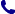• Contact
Choose interesting categories
I agree to receive promotional electronic communication(s).
Unsubscribe
• Institutional Clients
• Delivery
On-line access

# Bookstore

0.00 PLN
Bookshelf (0)

## Introduction to Discrete Mathematics via Logic and Proof

 Authors Publisher Springer, Berlin Year Pages 482 Version hardback Language English ISBN 9783030253578 Categories Discrete mathematics
\$48.89 (with VAT)
217.35 PLN / €46.60 / £40.45
Qty:
Delivery to United States

check shipping prices
Product to order
Delivery 5-6 weeks

## Book description

This textbook introduces discrete mathematics by emphasizing the importance of reading and writing proofs. Because it begins by carefully establishing a familiarity with mathematical logic and proof, this approach suits not only a discrete mathematics course, but can also function as a transition to proof. Its unique, deductive perspective on mathematical logic provides students with the tools to more deeply understand mathematical methodology-an approach that the author has successfully classroom tested for decades.

Chapters are helpfully organized so that, as they escalate in complexity, their underlying connections are easily identifiable. Mathematical logic and proofs are first introduced before moving onto more complex topics in discrete mathematics. Some of these topics include:

• Mathematical and structural induction
• Set theory
• Combinatorics
• Functions, relations, and ordered sets
• Boolean algebra and Boolean functions
• Graph theory

Introduction to Discrete Mathematics via Logic and Proof will suit intermediate undergraduates majoring in mathematics, computer science, engineering, and related subjects with no formal prerequisites beyond a background in secondary mathematics.

Introduction to Discrete Mathematics via Logic and Proof801 777 223